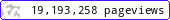•••••MadAsMaths.com :: I.Y.G.B. Practice Papers :: Special & Extension Papers :: SPX Practice Papers

I.Y.G.B. SPX PRACTICE PAPERS

These Extension Special Practice Papers follow the Mathematics/Further Mathematics non applied Syllabi used in recent years.
The aim of these papers is to give extremely able students the very best in difficulty.

Note that the solutions to these papers are available on request, however a maximum of 3 sets of solutions can be provided per request, and no repeated requests.

 spx_a.pdfspx_a_solutions.pdfspx_b.pdfspx_b_solutions.pdfspx_c.pdfspx_c_solutions.pdfspx_d.pdfspx_d_solutions.pdfspx_e.pdfspx_e_solutions.pdfspx_f.pdfspx_f_solutions.pdfspx_g.pdfspx_g_solutions.pdfspx_h.pdfspx_h_solutions.pdfspx_i.pdfspx_i_solutions.pdfspx_j.pdfspx_j_solutions.pdfspx_k.pdfspx_k_solutions.pdfspx_l.pdfspx_l_solutions.pdfspx_m.pdfspx_m_solutions.pdfspx_n.pdfspx_n_solutions.pdfspx_o.pdfspx_o_solutions.pdfspx_p.pdfspx_p_solutions.pdfspx_q.pdfspx_q_solutions.pdfspx_r.pdfspx_r_solutions.pdfspx_s.pdfspx_s_solutions.pdfspx_t.pdfspx_t_solutions.pdfspx_u.pdfspx_u_solutions.pdfspx_v.pdfspx_v_solutions.pdfspx_w.pdfspx_w_solutions.pdfspx_x.pdfspx_x_solutions.pdfspx_y.pdfspx_y_solutions.pdfspx_z.pdfspx_z_solutions.pdf